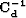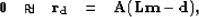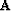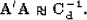Next: A modeling approach Up: Proposed solutions to attenuate Previous: Proposed solutions to attenuate

## A filtering method

The filtering method is motivated by the need for estimating the noise covariance operator when the data are noisy. The noise covariance operator has deep theoretical roots for least-squares estimation but its estimation remains somewhat obscure or difficult to achieve. This is why in practice, this matrix is approximated with diagonal operators. In this section I give a method that computes non-diagonal covariance matrices using prediction-error filters.

When coherent noise is present in the data, residual variables are no longer IID. The coherent noise will add color'' to the spectrum of the residual. The key idea is to recognize that the goal of the inverse covariance matrixis to absorb this spectrum. Now, as Claerbout and Fomel (2002) assert:

Clearly, the noise spectrum is the same as the data covariance only if we accept the theoretician's definition that E(d)=Fm. There is no ambiguity and no argument if we drop the word variance'' and use the word spectrum''.
This statement is the basis of the filtering method. It says that the data residuals (squared) should be weighted by their inverse multivariate spectrum for optimal convergence. Because a prediction-error filter (PEF) whitens data from which it was estimated Burg (1975), it approximates the inverse power spectrum. Thus a PEF (squared) with the inverse spectrum of the coherent noise accomplishes the role of the inverse covariance matrixin equation (). The fitting goals in equation () become, omitting the regularization term,(19)
whereis a PEF that whitens the coherent noise. Therefore, the noise covariance matrix is approximated as follows:(20)
This method is solely based on the whitening properties of the PEFs; other types of filters can be used as well. The next section introduces a noise modeling approach. I show that this method reduces to a weighting of the residual with a projection filter.Next: A modeling approach Up: Proposed solutions to attenuate Previous: Proposed solutions to attenuate
Stanford Exploration Project
5/5/2005